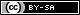# Dev Blog

 ./dev Original theme by orderedlist (CC-BY-SA) Where applicable, all content is licensed under a CC-BY-SA.## GCode Conversion Tools

At one point, I had good success with an Inkscape plugin called Gcodetools but it seems to have succumbed to bit-rot and doesn't work on my current Ubuntu installation (16.04).

I'm settling on a rough toolchain that takes some base format (PostScript/PDF/SVG/etc.), converts to "GNUPlot format" then converts to GCocde.

## Basic Workflow

• Orig -> SVG Create object in whatever tool and export to SVG
• SVG -> PS Use rsvg-convert to convert from SVG to PostScript
• PS -> GP Use pstoedit to convert to "gnuplot" polygon format
• GP -> GCode Order the polygons properly, removing duplicate boundaries and convert to GCode using clipcli, convert from GNUPlot format to GCode using gp2ngc and then rescale using other cli GCode tools.

## Installation

Some tools of relevance are:

Under Ubuntu, some of the tools can be installed via:

sudo apt-get install pstoedit librsvg2-bin


## Conversion

Though this is pretty hodge-podge, there are a few things to consider:

• pstoedit loses units when converting to RS274 GCode. I believe this only considers PostScript with "pixel" units, regardless of original units, then converts a pixel to 1/72 inches. A post scale has to be done if using pstoedit to rescale to the appropriate units
• Even if pstoedit is used, this creates a problem when trying to cut out shapes in the correct order. clipcli has an option to print out polygons in 'tree' order which should print the inner polygons first.
• I'll be using some of the tools that I've created below to rescale/etc. but in theory anything could be used, including (maybe the more standard and robust?) grecode as linked above.

The following is an example script to convert an input SVG file into GCode:

inpsvg="$1" sf=echo '72/25.4' | bc -l premul=echo 1000000 | bc -l invmul=echo "1/$premul" | bc -l

frapid=""
fslow="F800"
S="1.0"

if [[ "$inpsvg" == "" ]] ; then echo "provide input svg" exit 1 fi rawtype=file$inpsvg
checktype=file -b $inpsvg | cut -f1 -d' ' if [[ "$checktype" != "SVG" ]] ; then
echo -e "file $inpsvg is of type:\n\n$rawtype\n\nNnot an SVG file? Exiting.\n"
exit 1
fi

bn=basename $inpsvg .svg # causes duplicate paths otherwise # sed -i 's/fill="[^"]*"/fill="none"/g'$inpsvg

echo "creating $bn.ps" rsvg-convert -f ps -o$bn.ps $inpsvg pstoedit -f gnuplot$bn.ps $bn.gp clipcli -s$bn.gp -F -x $premul -T >${bn}-ord.gp

sfx_slow="$frapid S$S"
sfx_rapid="$fslow S0" echo gp2ngc -i${bn}-ord.gp -s "$invmul" --sfx-rapid "$sfx_rapid" --sfx-slow "$sfx_slow" -o${bn}.ngc
gp2ngc -i ${bn}-ord.gp --sfx-rapid "$sfx_rapid" --sfx-slow "$sfx_slow" | ngc_scale -s "$invmul" > ${bn}.ngc  ## Misc. In theory, pstoedit can be used to create GCode but pstoedit converts to the RS274 standard. Among other things, the RS274 includes variables so a substitution step needs to be involved in order to "normalize" to something that other GCode interpreters can understand (for example, the smoothieboard or grbl). There's still the problem of polygon ordering but assuming that's not an issue, the following is a "hacky" script does the substitution (no nested expressions, no non-trivial functions, run at your own risk): #!/usr/bin/python # # regexp substitution of variables. # Uses Python's "eval" to evaluate interior # after variable substitution. # # AGPLv3 license # import sys import re var_map = {} # variable decleration # var_decl_pat = re.compile( r'\s*#(\d+)\s*=\s*([^\s]+)\s*($[^$]*\))?\s*$' )

# not [], [], not []
#
expr_pat = re.compile( r'([^$]*)\[([^$]*)\]([^\[]*)' )

# not #*, #\d+, not #*
#
var_sub_pat = re.compile( r'([^#]*)(#\d+)([^#]*)' )

# consider comments separately to avoid matching '#' and
# other special characters
#
comment_pat = re.compile( r'$[^$]*\)' )

line_no = 0
for line in sys.stdin:
line_no += 1

line = line.rstrip()
for (comment) in re.findall(comment_pat, line):

line = re.sub(comment_pat, '', line)
m = re.match(var_decl_pat, line)
if m:
var_map[ "#" + str(m.group(1)) ] = str(m.group(2))
continue

varsub_line = ""
for (pfx, var_subs, sfx) in re.findall(var_sub_pat, line):
if var_subs in var_map:
pass
else:
print " ERROR on line", line_no, ", no variable mapping for", var_subs
sys.exit(1)
continue

varsub_line += pfx
varsub_line += var_map[var_subs]
varsub_line += sfx

if varsub_line == "":
varsub_line = line

xpr_match = re.search(expr_pat, varsub_line)
if not xpr_match: